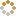﻿ N-B-U-T Online Judge :: NBUT 2015 Timed NOJ Training #003 :: Find MaxXorSum
• ### [C] Find MaxXorSum

• 时间限制: 2000 ms　内存限制: 65535 K
• 问题描述
• Given n non-negative integers, you need to find two integers a and b that a xor b is maximum. xor is exclusive-or.
• 输入
• Input starts with an integer T(T <= 10) denoting the number of tests.
For each test case, the first line contains an integer n(n <= 100000), the next line contains a1, a2, a3, ......, an(0 <= ai <= 1000000000);
• 输出
• For each test case, print you answer.
• 样例输入
• ```2
4
1 2 3 4
3
7 12 5```
• 样例输出
• ```7
11```
• 提示
• `无`
• 来源
• `Alex@NBUT`
• 操作
﻿